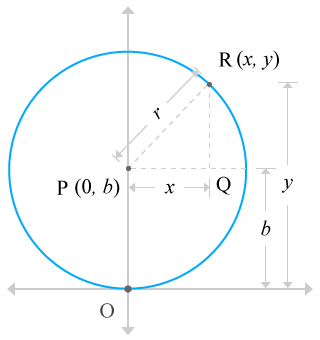# Equation of the Circle when circle passes through the origin and centre of the circle lies on y-axis

Expressing a circle in terms of a mathematical form expression when the circle passes through the origin of the Cartesian coordinate system and the centre of the circle lies on the $y$-axis of the Cartesian coordinate system is defined equation of the circle when the circle passes through the origin and centre of the circle lies on the $y$-axis.

A circle can be passed through the origin of the Cartesian coordinate system and also the centre of the circle can also lie on the $y$-axis of the Cartesian coordinate system. It can be written in mathematical form by understanding the relation of the circle with the Cartesian coordinate system and the equation of the circle exactly reveals how exactly the circle is in the Cartesian coordinate system.

## Derivation

Imagine a circle in the Cartesian coordinate system and assume it passes through the origin of the Cartesian coordinate system by having a radius of $r$ units and also assume the centre of the circle lies on the vertical $y$-axis.Assume, the centre of the circle is called as point $P$. It lies on the vertical $y$-axis. So, the distance from origin to centre of the circle in horizontal $x$-axis direction is zero units. Assume, the distance from the origin of the coordinate geometric system to centre of the circle is $b$ units. So, the coordinates of the centre of the circle is $P\left(0,b\right)$.

Consider a point on the circle and assume it represents all the points on the circle. Assume the variable point is located at $x$ and $y$ units distance from the origin in horizontal and vertical axis directions. It is assumed to call point $R$ and coordinate of this point is $R\left(x,y\right)$.

Draw a perpendicular line from point $P$ and that should be parallel to the $x$-axis. Similarly, draw a line from point $R$ and it should be perpendicular to the $x$-axis. These two lines are intersected at a point, called $Q$. Thus, a right angled triangle $\Delta RPQ$ is constructed.

The right angled triangle consists of three sides and the relation between them can be expressed in mathematical form according to Pythagorean Theorem.

${RP}^{2}={PQ}^{2}+{RQ}^{2}$

The length of the every side of the right angled triangle can be evaluated from the right angled triangle $\Delta RPQ$.

• Length of the hypotenuse is $PR=r$.
• Length of the adjacent side is $PQ=x$.
• Length of the opposite side is .

Substitute lengths of all three sides in the mathematical form relation, derived from the Pythagorean Theorem.

${r}^{2}={x}^{2}+{\left(y–b\right)}^{2}$

$⇒{x}^{2}+{\left(y–b\right)}^{2}={r}^{2}$

The circle is passing through the origin. So, the distance from the origin to centre of the circle is $OP$, known radius of the circle. In fact, the length of the $OP$ is already assumed $b$ units. It means $OP=b=r$. So, substitute $b$ instead of $r$ to get the simplified equation.

This algebraic expression is required mathematical form equation which represents an equation of a circle when the centre of the circle lies on the vertical axis and the circle passes through the origin of the Cartesian coordinate system.

Latest Math Topics
Latest Math Problems
##### Recommended Math Concepts###### How to derive equation of a Circle when the circle passes through the origin###### How to develop equation of a Circle when the Circle touches x-axis###### How to derive equation of a Circle when the Circle touches y-axis###### How to develop equation of a Circle when the Circle touches both axes###### How to derive Equation of the Circle when centre of the circle coincides with the origin###### How to develop Equation of the Circle when Circle passes through the origin and Centre of the Circle lies on x-axis###### How to develop Equation of the Circle when Circle doesn’t passes through the origin and Centre of the Circle lies on x-axis###### How to develop Equation of the Circle when Circle doesn’t passes through the origin and Centre of the Circle lies on y-axis###### How to develop Equation of a Circle in Standard form

A best free mathematics education website for students, teachers and researchers.

###### Maths Topics

Learn each topic of the mathematics easily with understandable proofs and visual animation graphics.

###### Maths Problems

Learn how to solve the maths problems in different methods with understandable steps.

Learn solutions

###### Subscribe us

You can get the latest updates from us by following to our official page of Math Doubts in one of your favourite social media sites.